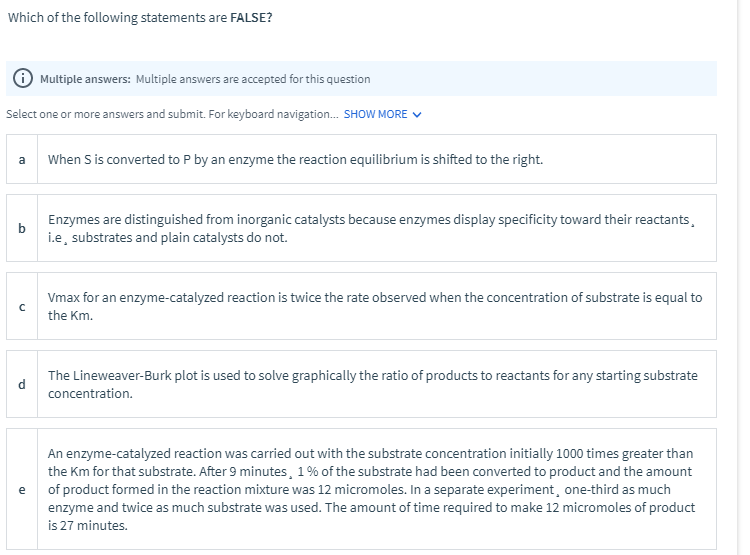# Which of the following statements are FALSE?

Discipline: Chemistry

Paper Format: APA

Pages: 1 Words: 275

Question

Which of the following statements are FALSE? Multiple answers: Multiple answers are accepted for this question Select one or more answers and submit. For keyboard navigation... SHOW MORE a When S is converted to P by an enzyme the reaction equilibrium is shifted to the right. b Enzymes are distinguished from inorganic catalysts because enzymes display specificity toward their reactants, i.e, substrates and plain catalysts do not. с Vmax for an enzyme-catalyzed reaction is twice the rate observed when the concentration of substrate is equal to the Km. d The Lineweaver-Burk plot is used to solve graphically the ratio of products to reactants for any starting substrate concentration. An enzyme-catalyzed reaction was carried out with the substrate concentration initially 1000 times greater than the Km for that substrate. After 9 minutes, 1% of the substrate had been converted to product and the amount e of product formed in the reaction mixture was 12 micromoles. In a separate experiment, one-third as much enzyme and twice as much substrate was used. The amount of time required to make 12 micromoles of product is 27 minutes.#(a): An enzyme catalyst only helps to reach the equilibrium faster without disturbing the equilibrium cosntant. Since equilibrium constant remains same, the equilibrium is not shifted by an enzyme. Hence False.

#(b): Enzymes are special due to their ability to be specific towards a single reactant or substrate due their ability to identify only the substrate that are specific to them. On the other hand this property is not found in a plane inorganic catalyst and substrates. Hence True.

# (c): According to Michaelis-Menten kinetics, the formula for Vmax of an enzyme catalyzed reaction is:

V = Vmax * [S] / (Km + [S]) ----- (1)

where V = observed rate

[S] = Substrate concentration

Given Km = [S] ------ (2)

From equation-(1) and (2), replacing [S] by Km

=> V = Vmax * [S] / (Km + [S])

=> V = Vmax * Km / (Km + Km)

=> V = Vmax * Km / 2Km

=> V = Vmax / 2 [Km cancels out from numerator and denominator]

=> Vmax = 2*V

Hence Vmax is twice the rate observed. Hence True.

#(d): Lineweaver-Burk plot of graphical representation of how the reciprocal of reaction rate(1/V) varies with the reciprocal of substrate concentration (1/[S]). The slope of the equation is the ratio of Km and Vmax i.e slope = Km/Vmax. Hence we can find the ratio of product to reactants using the slope of Lineweaver-Burk plot.

Hence True.

#(e): An enzyme helps in reaching the reaction faster. So smaller the enzyme concentration larger is the time required to achieve product. Since on the separate experiment, we have decreased the enzyme concentration by 1/3rd, the time taken to form the same amount of product (12 micromoles) will be 3 times higher.

Hence total time required = 9*3 = 27 minutes.

Hence True

Hence only option (a) is False and rest are True.